# Conditional distribution

A function of an elementary event and a Borel set, which for each fixed elementary event is a probability distribution and for each fixed Borel set is a conditional probability.

Let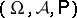be a probability space,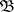the-algebra of Borel sets on the line,a random variable defined on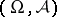anda sub--algebra of. A function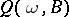defined onis called a (regular) conditional distribution of the random variablewith respect to the-algebra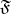if:

a) for fixed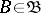the functionis-measurable;

b) with probability one, for fixedthe functionis a probability measure on;

c) for arbitrary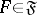,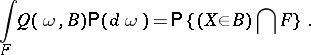Similarly one can define the conditional distribution of a random elementwith values in an arbitrary measurable space. If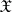is a complete separable metric space andis the-algebra of Borel sets, then the conditional distribution of the random element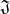relative to any-algebra,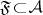, exists.

The function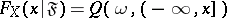is called the conditional distribution function of the random variablewith respect to the-algebra.

The conditional distribution (conditional distribution function) of a random variablewith respect to a random variableis defined as the conditional distribution (conditional distribution function) ofwith respect to the-algebra generated by.

The conditional distribution function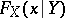of a random variablewith respect tois a Borel function of; for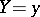its value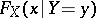is called the conditional distribution function offor a fixed value of. Ifhas a density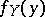, then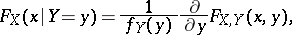where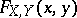is the joint distribution function ofand.

How to Cite This Entry:
Conditional distribution. V.G. Ushakov (originator), Encyclopedia of Mathematics. URL: http://encyclopediaofmath.org/index.php?title=Conditional_distribution&oldid=11344
This text originally appeared in Encyclopedia of Mathematics - ISBN 1402006098